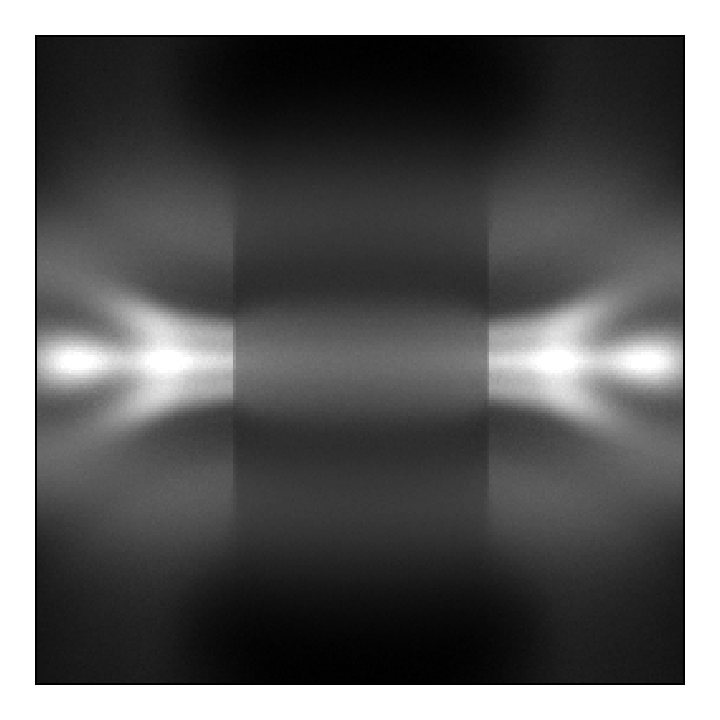Atomic Data¶

Name

Description

Preview

Photon Emissivity Coefficients

Sampling and plotting PEC rates provided by OPEN-ADAS.Beam-Plasma Interaction Rates

Sampling and plotting various beam-plasma interaction rates provided by OPEN-ADAS.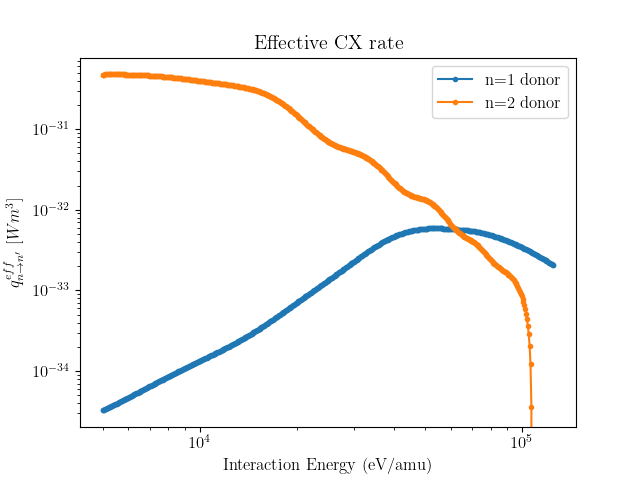Fractional Abundances

Sampling and plotting Neon fractional abundances with the ADAS subscription package.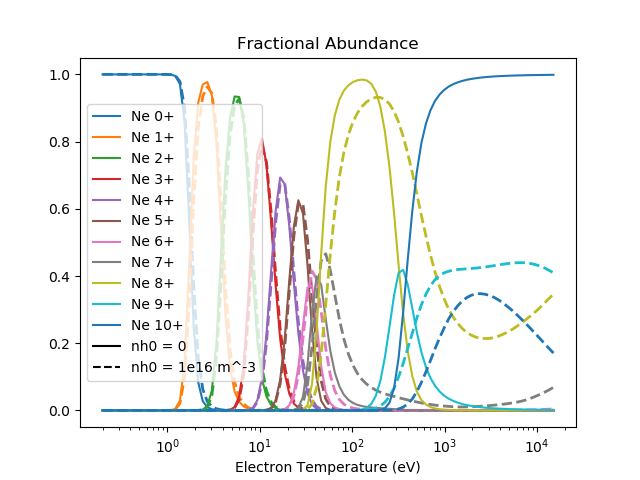Sampling and plotting total and stage resolved radiated powers with the ADAS subscription package.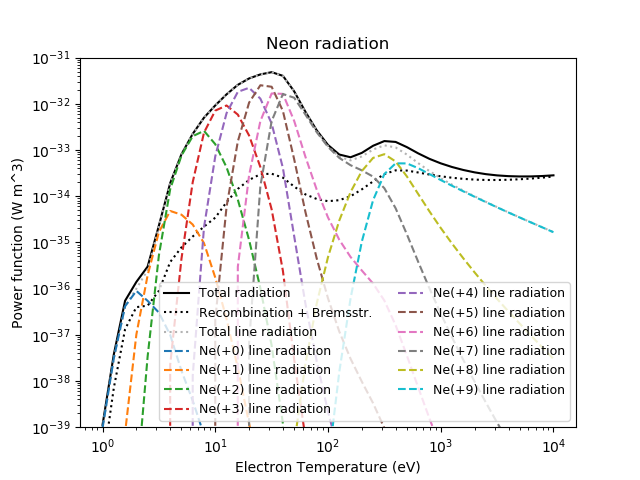Creating Plasmas¶

Name

Description

Preview

Analytic Functions

Specifying plasma distributions with analytic functions.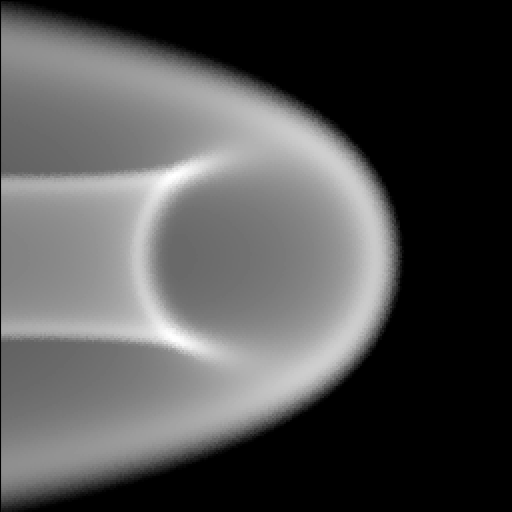Flux Function Plasmas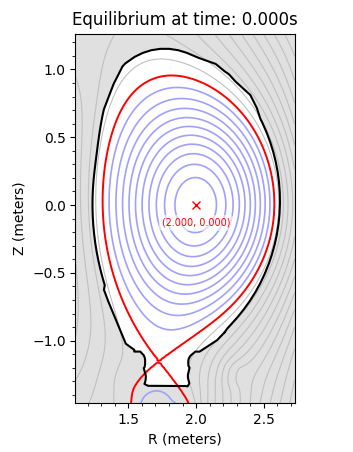2D Mesh Plasmas

Specifying a plasma distribution with a 2D r-z triangular mesh.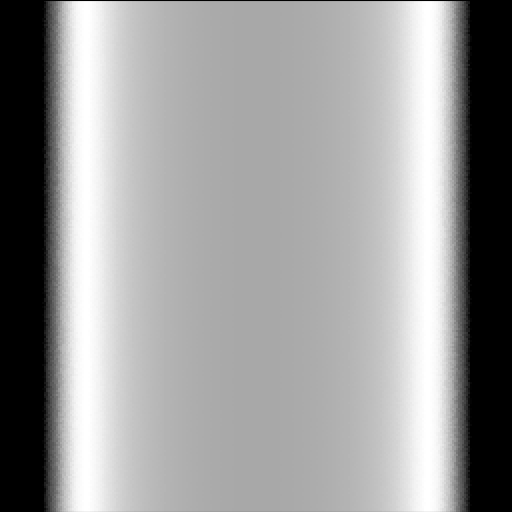Slab Plasma

Constructing a slab of plasma defined along +ve x.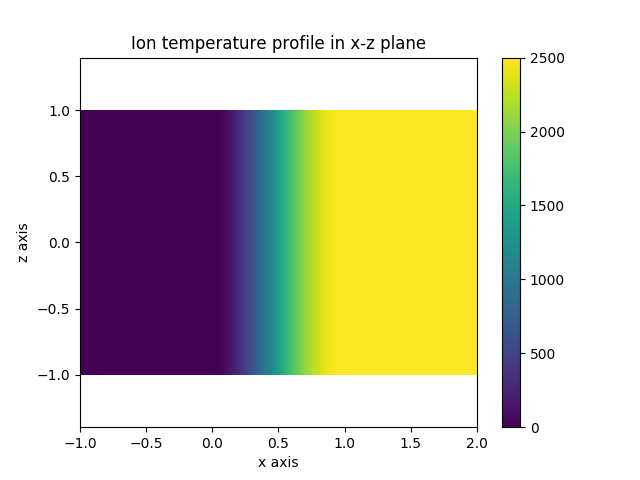Beams into Plasmas

Specifying a mono-energetic neutral beam that interacts with a plasma.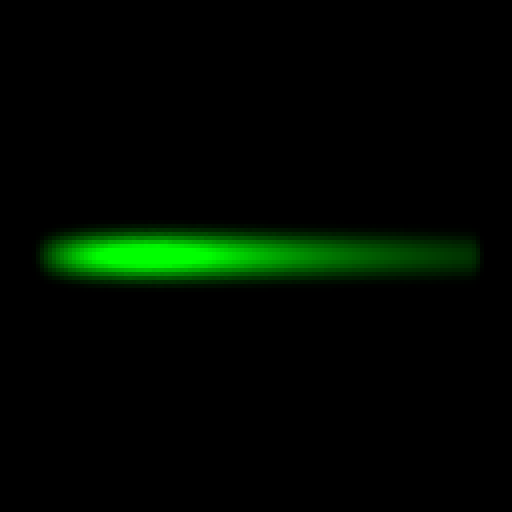Name

Description

Preview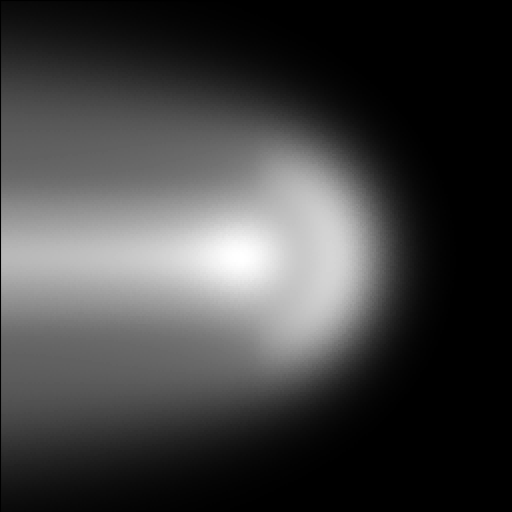Defining A Wall From A 2D Polygon

A toroidal mesh representing the tokamak wall is made from a 2D polygon outline.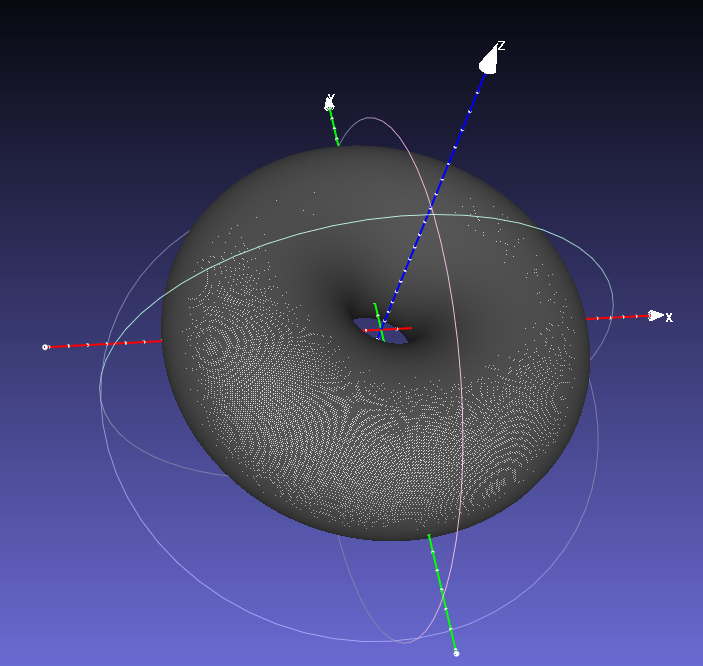Calculating the power load by exploiting symmetry. We manually create an array of detectors for sampling.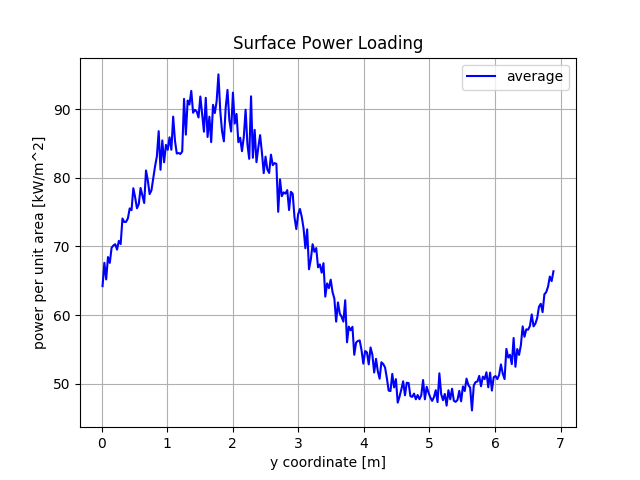Mesh Observer

Calculating powers on an arbitrary 3D surface (Raysect docs).An older demonstration of the tutorials above using a SOLPS simulation and an AUG wall outline.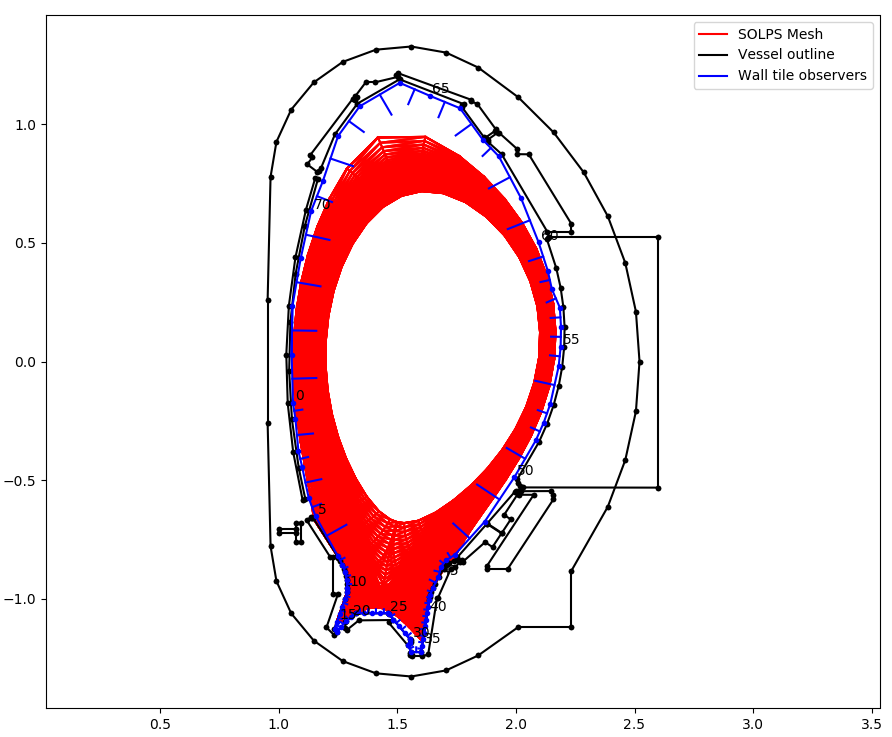Active Spectroscopy¶

Name

Description

Preview

Charge Exchange Spectroscopy (CXS)

A simple CXS model for a beam into a plasma slab.Beam Emission Spectroscopy

A simple Beam Emission Spectroscopy (BES) model for a beam into a plasma slab.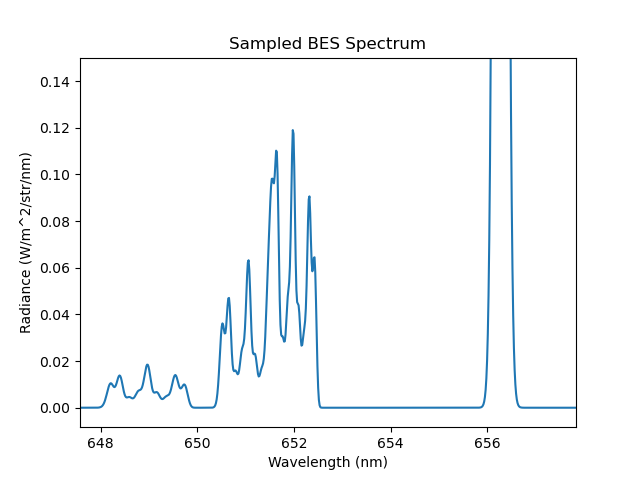Passive Spectroscopy¶

Name

Description

Preview

Impact Excitation and Recombination

Modelling background lines due to electron impact excitation and recombination.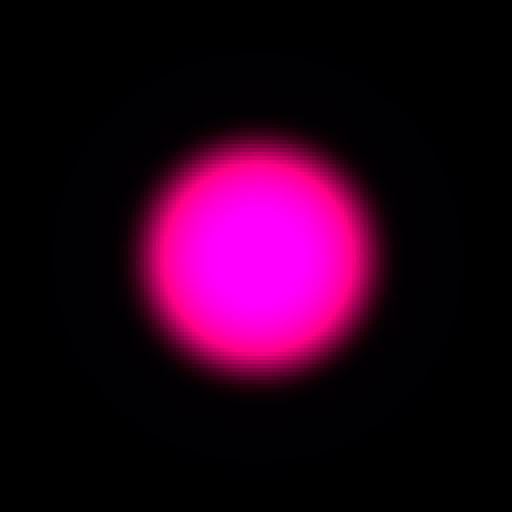Multiplet Line Ratios

Including experimentally determined multiplet line ratios.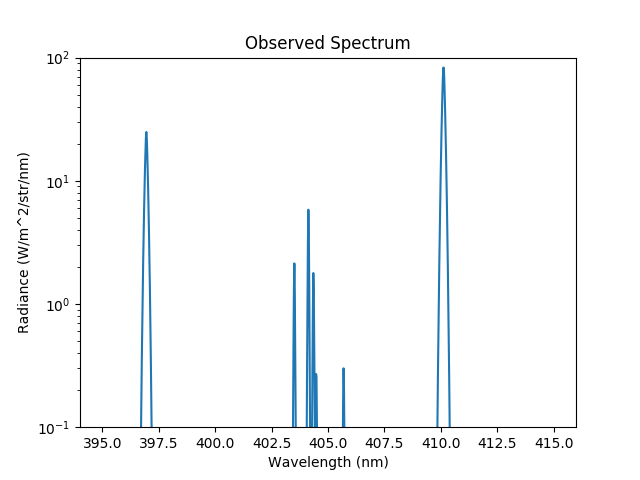Zeeman Spectroscopy

Specifying a Zeeman triplet or multiplet line shapes.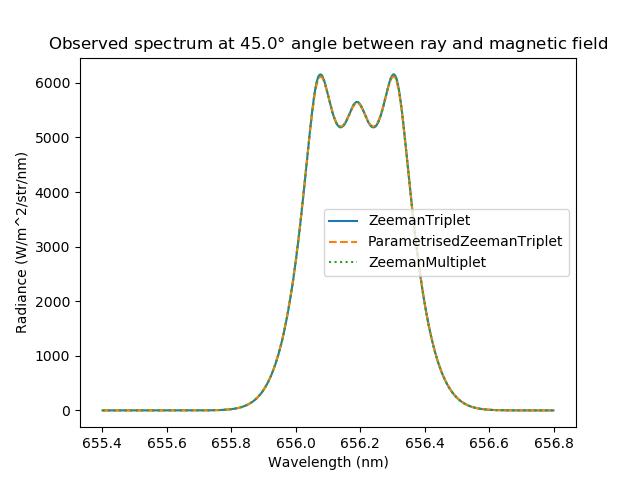Bolometry¶

Name

Description

Preview

Camera From Primitives

Designing a simple bolometer camera using raysect primitivesCamera From Mesh and Coordinates

Designing a simple bolometer camera using a mesh file and vertex coordinatesCalculating the measured power and radiance on bolometer from a 3D radiation functionCalculating the Etendue

Calculating the etendue of a bolometer detectorGeometry Matrix Calculation Using Voxels

Calculating the geometry matrix for a bolometer system using the voxel framework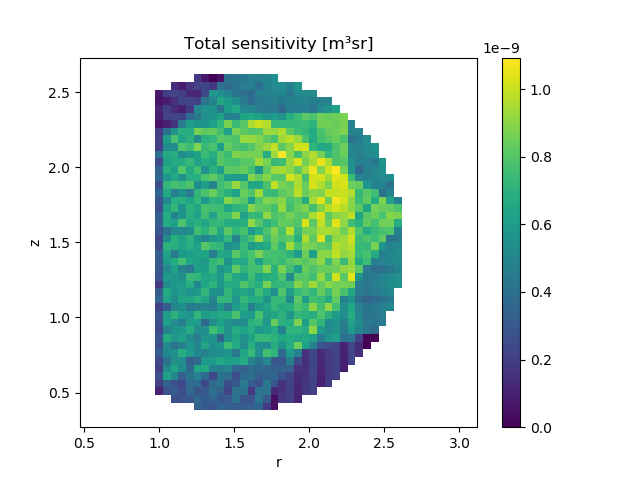Bolometer Inversions Using Voxels

Performing inversions using geometry matrices and the voxel framework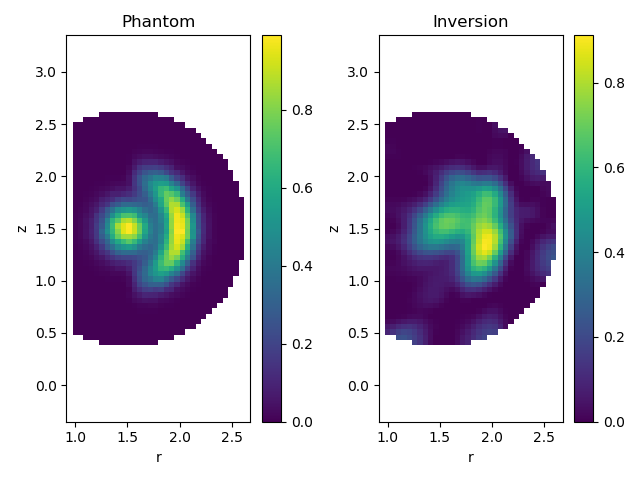Geometry Matrix Calculation Using Ray Transfer Objects

Calculating the geometry matrix for a bolometer system using ray transfer objects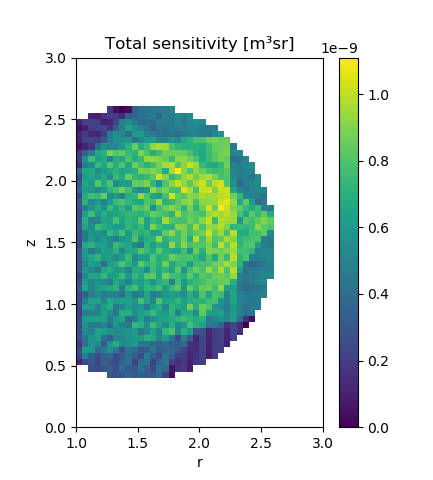Bolometer Inversions Using Ray Transfer Objects

Performing inversions using geometry matrices from a ray transfer object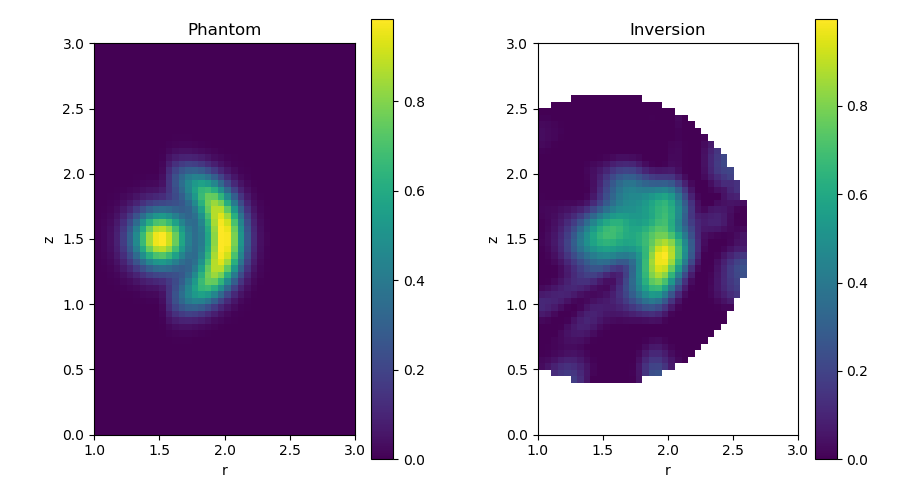Geometry Matrices¶

Name

Description

Preview

Rectangular regular grid

Calculating the geometry matrix for a rectangular emitter defined on a regular grid.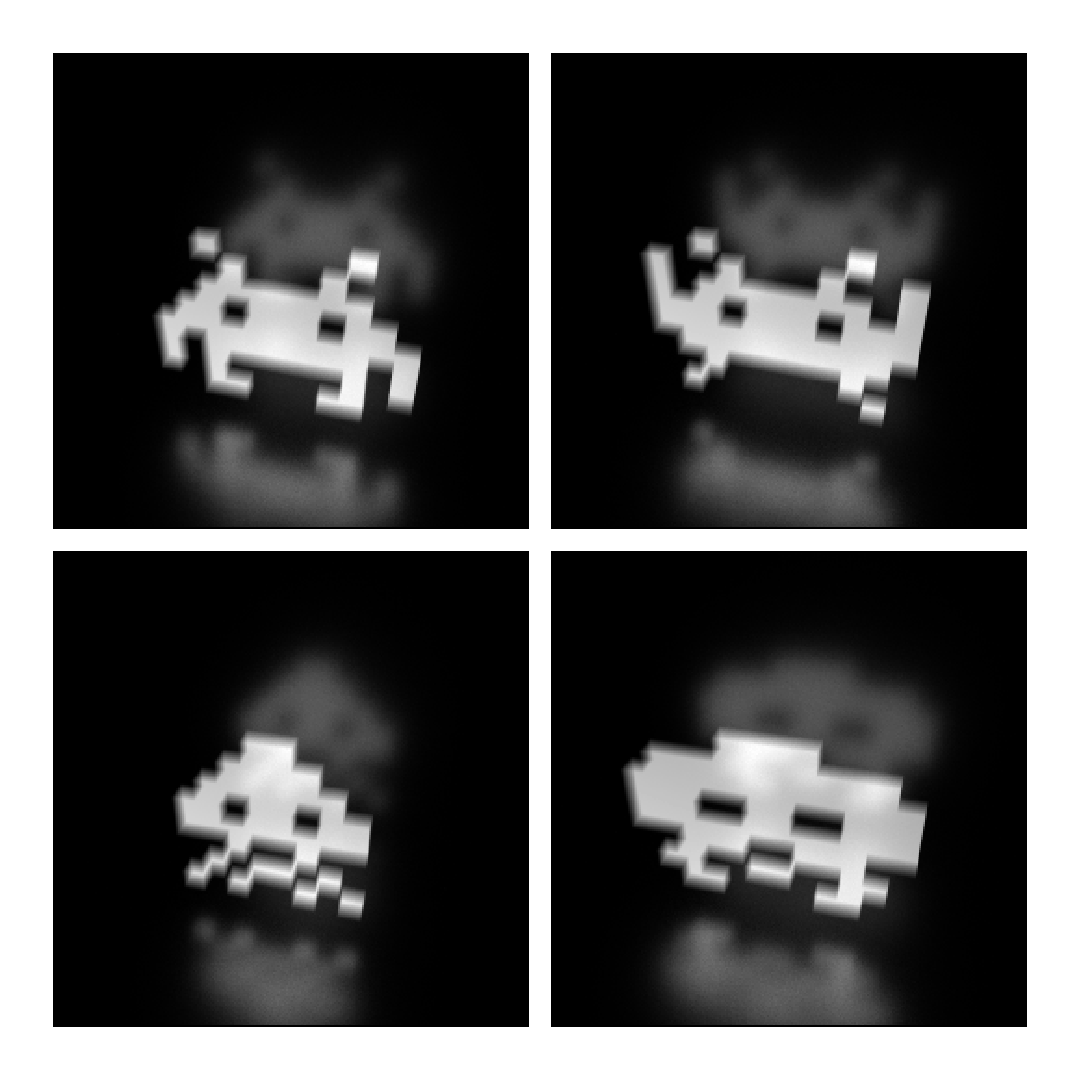Cylindrical regular grid

Calculating the geometry matrix for a cylindrical emitter defined on a regular grid.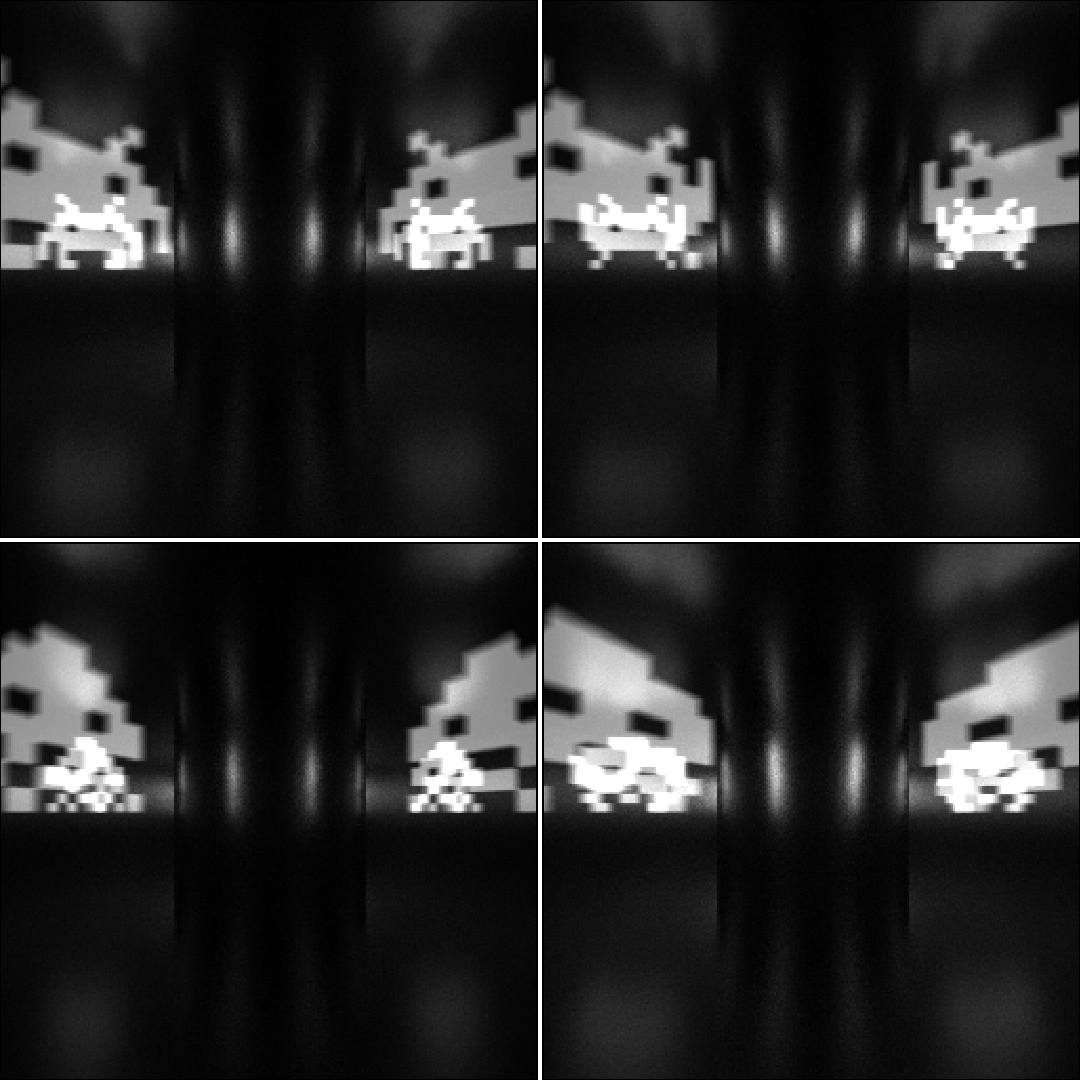Axisymmetrical (toroidal) regular grid

Calculating the geometry matrix for an axisymmetrical toroidal emitter defined on a regular grid. Applying a mask to filter out extra grid cells.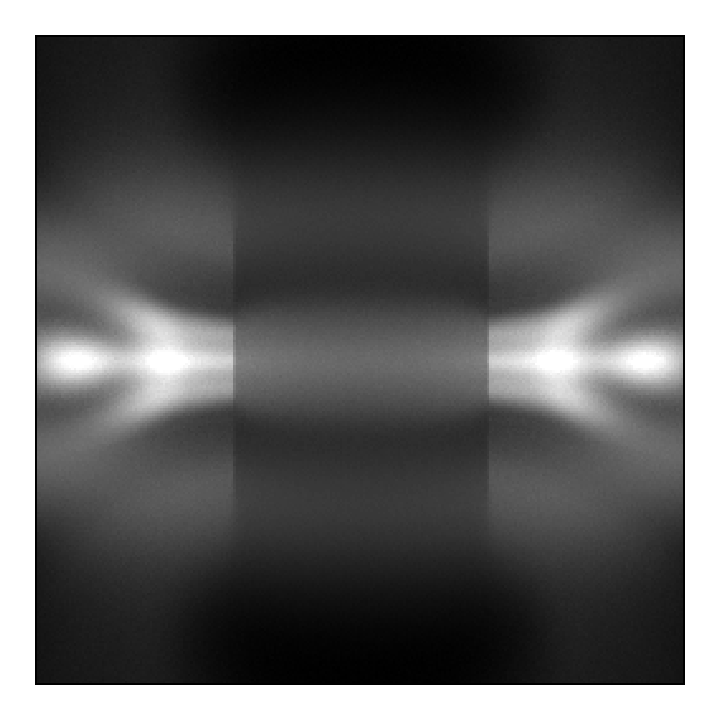Axisymmetrical (toroidal) regular grid with custom mapping of light sources

Mapping multiple grid cells into a single light source.